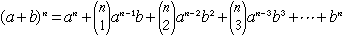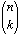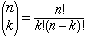Sender: "Howard E. Lutz" How do you find each successive numerical term in this equation y+dy=(x+dx)5 =x5+5*x4dx+10*x3(dx)2+10*x^2(dx)3+5*x(dx)4+(dx)5 I would appreciate an explanation of the method to find the numeric coefficient in a binomial expansion Thanking you in advance Hi Howard, In the binomial expansion of (a + b)n each term is a constant times akbn-k where the constant is n choose k. that isThe binomial coefficient n choose k, writtenis given bywhere for each integer n, n! = n(n-1)(n-2)...1. Thus, for example, 5! = 5x4x3x2x1 = 120. Cheers, Penny Go to Math Central